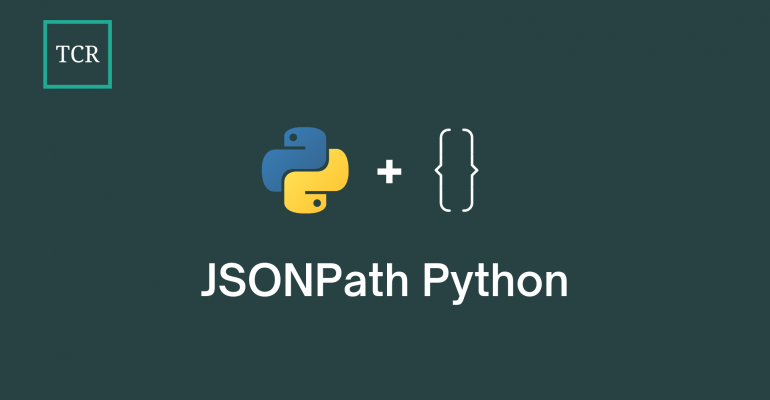Thanks to theidioms.com

## JSONPath Python – Examples and Usage in Python# JSONPath Python – Examples and Usage in PythonJSONPath Python is a way to parse JSON data through the use of pre-defined syntaxes in Python. It provides an extension to how you can work with JSON data in Python with fewer lines of code and less memory usage.

In this article, you will learn how to use JSONPath for Python along with a brief introduction to JSON data.

## What is JSON?

If you are unfamiliar with JSON data in general, JSON stands for JavaScript Object Notation and is an open standard file format and data interchange format. Here’s an example of JSON data:

``````{

"age": 27,
"city": "New York",
"state": "NY",
"postalCode": "10021-3100"
},
"phoneNumbers": [
{
"type": "home",
"number": "212 555-1234"
},
{
"type": "office",
"number": "646 555-4567"
}
],
"children": [],
"spouse": null
}``````

As you can see, working with such data in Python can be hard since there are multiple braces and brackets to work with. By using JSONPath for Python, you are able to parse such JSON data easily.

Let us learn how to get started with JSONPath for Python.

## Getting started with JSONPath for Python

To get started with JSONPath for Python, you can install the jsonpath-ng library using Python’s Package Manager (pip).

The jsonpath-ng library is a standard-compliant implementation of JSONPath for Python. This library differs from other JSONPath implementations in that it is a full language implementation, meaning the JSONPath expressions are first-class objects, easy to analyze, transform, parse, print, and extend.

To install the jsonpath-ng library, open up your command line/terminal and run the following command:

`pip install jsonpath-ng`

This will install the latest version of the jsonpath-ng library on your machine. You can then import it in Python using a Python IDE or Python Shell by writing the following line of code:

`import jsonpath_ng`

If running this line of code doesn’t give an error, then, you’ve successfully installed and imported jsonpath-ng in Python. Note that the jsonpath-ng library is written as `jsonpath_ng` in Python.

## JSONPath Python Example

Here’s an example of how JSONPath can be used in Python to work with JSON data:

```# Importing jsonpath-ng library in Python
import jsonpath_ng

# Initializing JSON data
json_data = {'foo': [{'baz': 1}, {'baz': 2}]}

# Setting up a parser
jsonpath_expr = jsonpath_ng.parse('foo[*].baz')

# Parsing the values of JSON data
list_val = [match.value for match in jsonpath_expr.find(json_data)]

# Printing the parsed values
print(list_val)```
`[1, 2]`

Here, we first set up a JSON parser by using the `parse()` method from the jsonpath-ng library. This parse method defines what we want to parse which is in this case the ‘baz’ keys.

Then, using the `find()` method, we find all of the matches in the JSON data and extract the value of each match.

You can also perform other kinds of operations using JSONPath for Python:

```# Importing jsonpath-ng library in Python
import jsonpath_ng

# Automatically providing ids for bits of data that do not have them
jsonpath_ng.jsonpath.auto_id_field = "id"
print(
[
match.value
for match in jsonpath_ng.parse("foo[*].id").find(
{"foo": [{"id": "bizzle"}, {"baz": 3}]}
)
]
)

# A handy extension: named operators like `parent`
print(
[
match.value
for match in jsonpath_ng.parse("a.*.b.`parent`.c").find(
{"a": {"x": {"b": 1, "c": "number one"}, "y": {"b": 2, "c": "number two"}}}
)
]
)```
```['foo.bizzle', 'foo.']
['number one', 'number two']```

The first print statement in the above JSONPath Python example provides ids for bits of data that do not have the given parse ‘key’ value, that is, ‘id’.

The second print statement in the above JSONPath Python example performs nested data extraction using the `parent` keyword.

## JSONPath Python Syntax – Complete List

The complete JSONPath Python Syntax for the jsonpath-ng library is as given below:

• Atomic expressions:
• JSONPath operators:
• Field specifiers:
• Array specifiers: